# RRB PO Quantitative Aptitude Quiz- 27

## RRB PO Prelims Quantitative Aptitude Quiz

Quantitative aptitude measures a candidate’s numerical proficiency and problem-solving abilities. It is the most important section of almost all competitive exams. Candidates are often stymied by the complexity of Quantitative Aptitude Questions but if they practice more and more questions it will become quite easy. So, here we are providing you with the RRB PO Quantitative Aptitude Quiz to enhance your preparation for your upcoming examination. Questions given in this RRB PO Quantitative Aptitude Quiz are based on the most recent and the latest exam pattern. A detailed explanation for each question will be given in this RRB PO Quantitative Aptitude Quiz. This RRB PO Quantitative Aptitude Quiz is entirely free of charge. This RRB PO Quantitative Aptitude Quiz will assist aspirants in achieving a good score in their upcoming examinations.

1. Two vessels A and B contain milk and water mixed in the ratio 5 : 3 and 2 : 3 respectively . When these mixtures are mixed to form a new mixture containing half milk and half water, so in what ratio they must be mixed?
(a) 2 : 5
(b) 3 : 5
(c) 4 : 5
(d) 7 : 3
(e) 5 : 3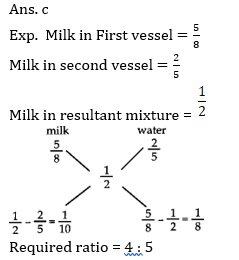2. Divide Rs 6000 into two parts, so that simple interest on the first part for 2 years at 6% per annum may be equal to the simple interest on the second part for 3 years at 8% per annum. Find the two parts?
(a) Rs 4000, Rs 2000
(b) Rs 5000, Rs 1000
(c) Rs 3000, Rs 3000
(d) Rs 3500, Rs 2500
(e) Rs 1500, Rs 45003. Shikha calculates his Profit % on SP whereas Ramesh calculates profit percent on CP. They find that the difference in their profit is Rs. 1000. If SP of Both are same and both gets 25% profit. Find their SP.
(a)Rs. 5000
(b)Rs. 4500
(c)Rs. 3000
(d) Rs. 2500
(e) None of the above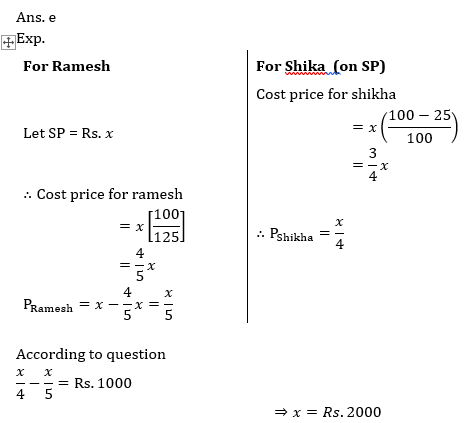4. A, B , C and D purchased a restaurant for Rs. 56 lakhs. The contribution of B, C and D together is 460% of A alone, while the contribution of A, C and D together is 366.66% to that of B’s contribution and the contribution of C is 40% of the contribution of A, B and D together. What is the amount contributed by D ?
(a) 10 lakhs
(b) 12 lakhs
(c) 16 lakhs
(d)18 lakhs
(e) None of these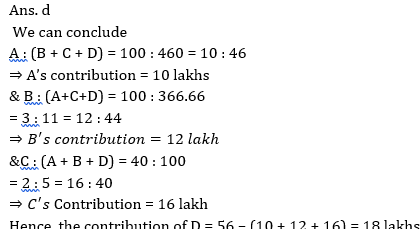5. What will be percentage profit after selling an article at a certain price if there is a loss of 25/2% when the article is sold at half of the previous SP ?
(a) 75%
(b) 80%
(c) 100%
(d) 85%
(e) 70%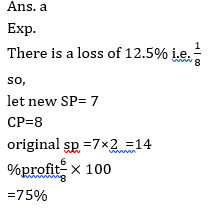Directions (6-10): In each of these questions, two equations (I) and (II) are given. You have to solve both the equations and give answer
6. I. 2×2 – 11x + 12 = 0
II. 3y2 – 16 y + 5 = 0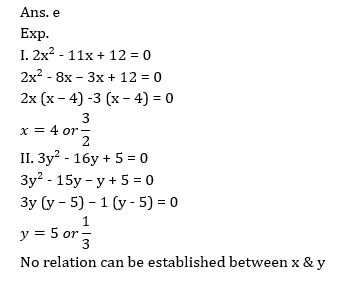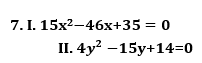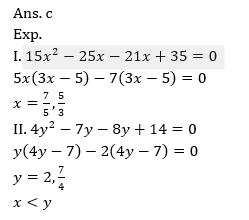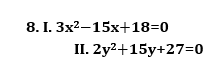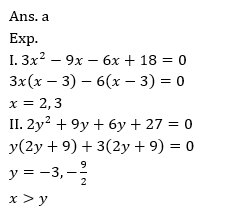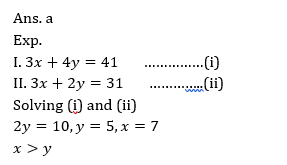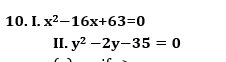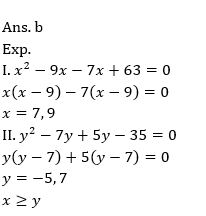###Click to Buy Bank MahaCombo PackageRecommended PDF’s for:

#### Most important PDF’s for Bank, SSC, Railway and Other Government Exam : Download PDF Now

AATMA-NIRBHAR Series- Static GK/Awareness Practice Ebook PDF Get PDF here
The Banking Awareness 500 MCQs E-book| Bilingual (Hindi + English) Get PDF here
AATMA-NIRBHAR Series- Banking Awareness Practice Ebook PDF Get PDF here
Computer Awareness Capsule 2.O Get PDF here
AATMA-NIRBHAR Series Quantitative Aptitude Topic-Wise PDF Get PDF here
AATMA-NIRBHAR Series Reasoning Topic-Wise PDF Get PDF Here
Memory Based Puzzle E-book | 2016-19 Exams Covered Get PDF here
Caselet Data Interpretation 200 Questions Get PDF here
Puzzle & Seating Arrangement E-Book for BANK PO MAINS (Vol-1) Get PDF here
3